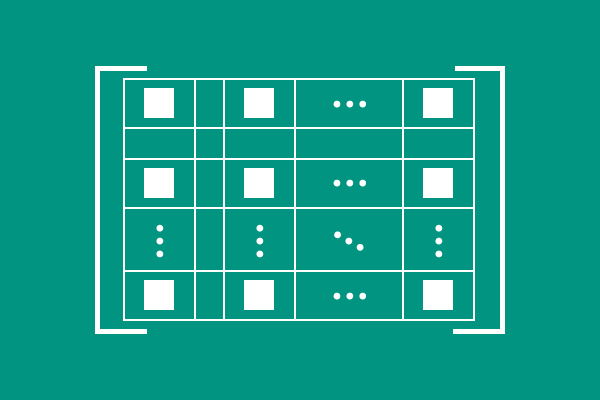# Elements of a Matrix

A quantity, displayed inside a matrix in a specific row and column is called an element of the matrix.The space inside a matrix is divided as required number of rows and columns on the basis of total number of elements. Every element is displayed in specific row and column but every two elements in a row or column are separated by some space.

#### Example

$3$, $6$, $-7$, $8$, $0$ and $4$ are elements in the following example matrix.

$\begin{bmatrix} 3 & 6 & -7 \\ 8 & 0 & 4 \\ \end{bmatrix}$

There is some space between every two elements in each row and each column for displaying them clearly in a matrix.

## Representation

Every element of a matrix is usually represented by a variable with two subscripts in which first subscript denotes the row of element and the second subscript denotes the column of the same element.

#### General form

All elements are displayed in a matrix in general form.

$\begin{bmatrix} e_{11} & e_{12} & e_{13} & \cdots & e_{1n} \\ e_{21} & e_{22} & e_{23} & \cdots & e_{2n} \\ e_{31} & e_{32} & e_{33} & \cdots & e_{3n} \\ \vdots & \vdots & \vdots & \ddots & \vdots \\ e_{m1} & e_{m2} & e_{m3} & \cdots & e_{mn} \\ \end{bmatrix}$

In this example, each element is displayed by a variable with two subscripts. The first and second subscripts of the literal represent row and column of the respective element.

1. The element $e_{11}$ represent an element belongs to first row and first column.
2. The element $e_{21}$ expresses an element belongs to second row and first column.
3. The element $e_{45}$ denotes an element belongs to fourth row and fifth column.

#### Compact form

A matrix can also be expressed in compact form. In this method, only one element is displayed by a variable with two subscripts but the two subscripts are also denoted by two variables.

If the row and column are denoted by $i$ and $j$ respectively, then the matrix is expressed in compact form as follows.

$\begin{bmatrix} e_{ij} \end{bmatrix}$

The element $e_{ij}$ represents every element in the matrix generally and $i = 1, 2, 3 \ldots$ and $j = 1, 2, 3 \ldots$

Latest Math Topics
Jun 26, 2023
Jun 23, 2023

Latest Math Problems
Jul 01, 2023
Jun 25, 2023
###### Math Questions

The math problems with solutions to learn how to solve a problem.

Learn solutions

Practice now

###### Math Videos

The math videos tutorials with visual graphics to learn every concept.

Watch now

###### Subscribe us

Get the latest math updates from the Math Doubts by subscribing us.